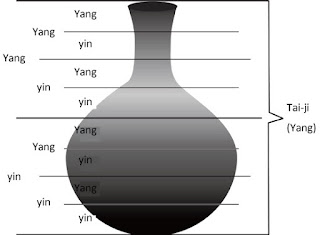## Saturday, June 15, 2013

### Mook (2): The Tai-ji (2)

Let us talk about the Tai-ji from the different perspective.

This is an illustration of the Earth and the solar system (Figure 1).

When we are focusing on the existence of the Earth, we can see the Earth as the Tai-ji, the fundamental yang, right? Then, what is the power supporting the Earth as the visible existence? That is the power called the gravity or the attractive force. Without it, this Earth can never exist.Figure 1

We focused on the Earth, so the Earth was the Tai-ji, but if we focus on the solar system this time, the whole solar system becomes the Tai-ji. Then, the power makes the solar system exist is also the gravitational force.

Let us focus on something more familiar with. This is the vase (Figure 2). Now we are looking at  this vase as the Tai-ji. The Tai-ji is yang, right. Then, how can this vase exist? This is also because there is a gravitational pull. If there is no gravitational power, the vase would become pieces, or we can see pieces, so it would become something less than pieces. That's what we understand.Figure 2

We can divide this Tai-ji into upper and lower, right? We can use the words yin and yang. Then, both the upper and lower parts can be divided into yin and yang as well. It can be subdivided forever.

Can you notice that the classified yin is different from the fundamental yin that is on the basis of the Tai-ji in terms of its meaning? Yin written in Figure 2 is the relative yin of the fundamental yang.

Next, when you focus on one of the relative yang, this yang is divided into yin and yang, in that case, the original yang becomes the Tai-ji (Figure 3).Figure 3

You might get an impression that yang is divided into yin and yang, and yin is divided into yin and yang, but in fact it is always that the Tai-ji is divided into yin and yang, and that's all. Are you confused now? The Tai-ji, yin and yang, the yin-yang concept consists of these three ideas only. You always replace the beginning with the Tai-ji. The Tai-ji is divided into yin and yang, and the yin and yang become the Tai-ji and each is divided into yin and yang, and the same thing can be repeated, right? Each time, there is a begining, and the beginning is treated as the Tai-ji. If this becomes clear, you can understand the main part of the Tai-ji / yin-yang theory roughly.

*This is translated from "Mook Shakuju Therapy" vol.1 (2013). This is the summary of Kobayashi sensei's lecture held in Chiba on February, 2012.